# Ship Detection in Satellite Images using Mask R-CNN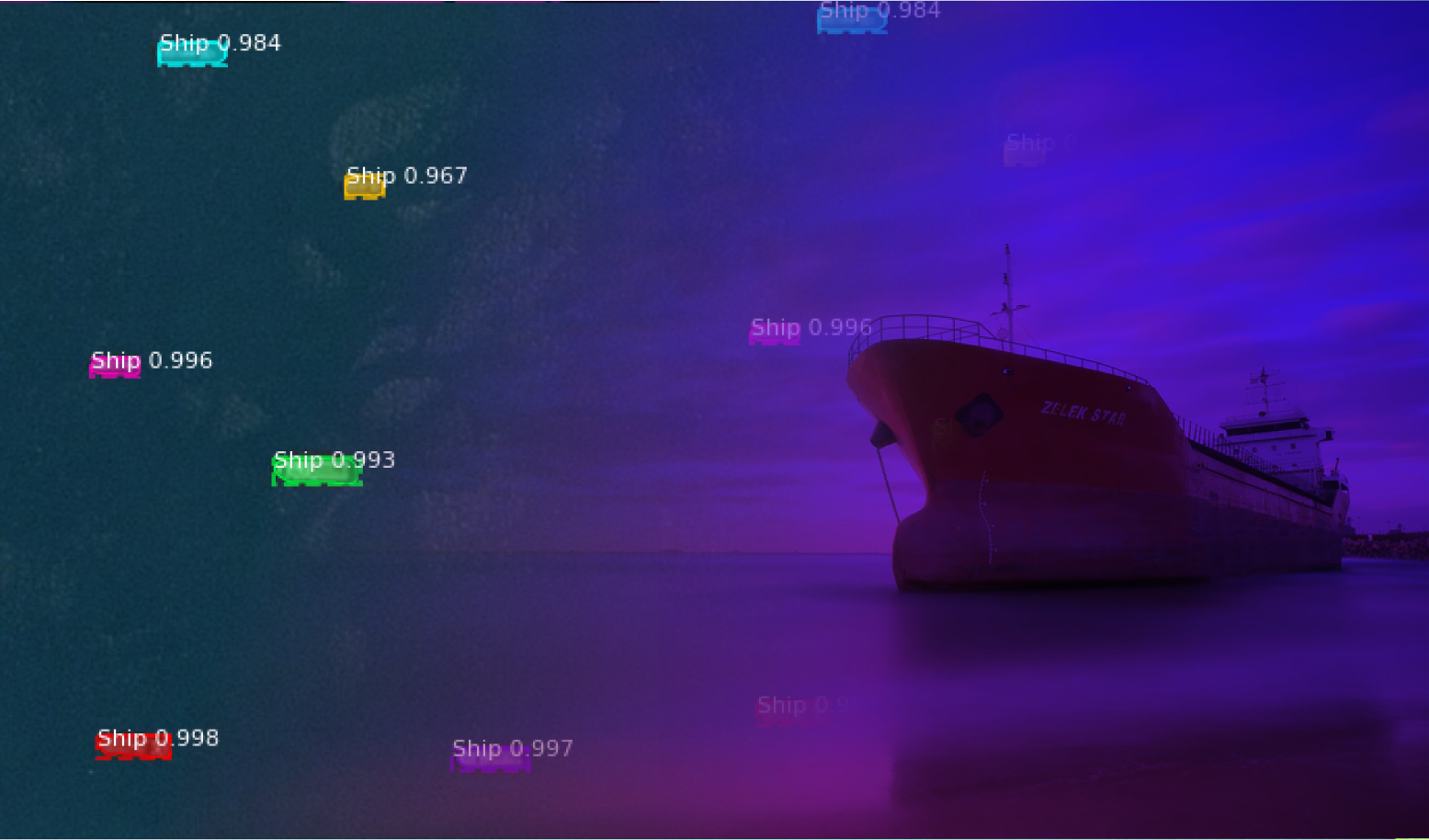Nowadays, with shipping traffic increasing at a rapid pace, ship detection from satellite images is crucial. The increase in movement of ships in the sea, chancing of ship accidents, and illegal activities have also compelled many organizations to keep a closer watch over the open seas.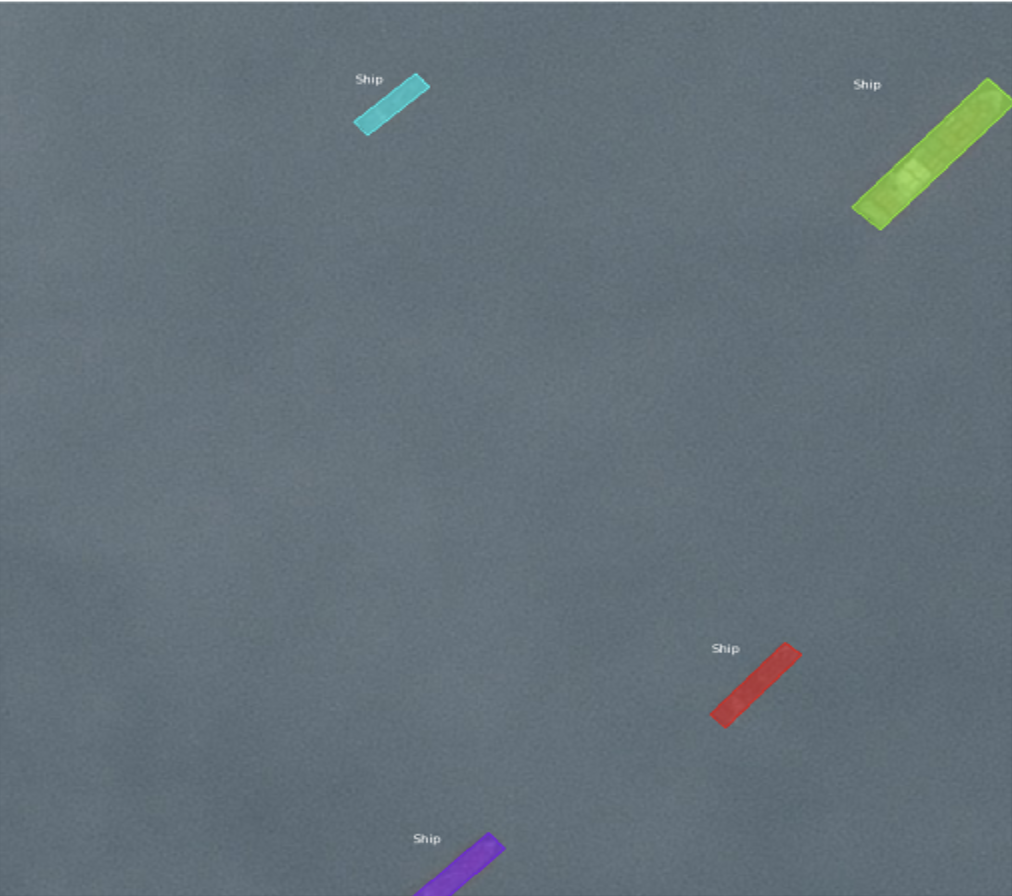In this article we will build an algorithm to automatically detect objects from satellite images and identify whether the detected objects are ships or not.

Since this is a deep learning problem, we will need more than the CPU power of a normal laptop and turn to a GPU system to implement an algorithm for it. Spell.ml is the fastest and most powerful end-to-end platform for Machine Learning and Deep Learning.

### Project Setup

We will upload our Jupyter notebook and run it in the GPU environment on Spell.ml.

Let’s learn how to run the process of training a model using Spell. First, we will start by setting up the workspace, and then run the Python notebook.

1. First, sign up to the Spell Web Panel https://spell.ml/ and log in to the web portal. For just signing up with Spell, you will get \$10 in free GPU credit!
2. Click on Workspace > Create Workspace and give a name to the workspace, such as Ship_Detection and continue. To the Add Code field, add the GitHub code link. All the code, Python notebook, and data will be picked from Github and then click Continue.
3. In Environment, select Machine Type to V100. V100 is a type of GPU. Set Framework as Default, Select Jupyter Notebooks, Set Pip Packages as scikit-image,keras==2.2.0 and leave other entries, and then click Continue.
It will take us to the Resources Page. In resources→ uploads, upload mask_rcnn_coco.h5 weights files and then mount it by clicking the checkbox and then click continue.
4. Now, on the next window, all workspace details will appear. Cross verify all details. Click back in case you need to make any changes.
5. Now, the server will be starting with server logs.
6. When the server runs successfully below, a Jupyter window will appear. All the data and Jupyter notebook from the Github profile will appear here.
7. Open the Jupyter notebook and run the code.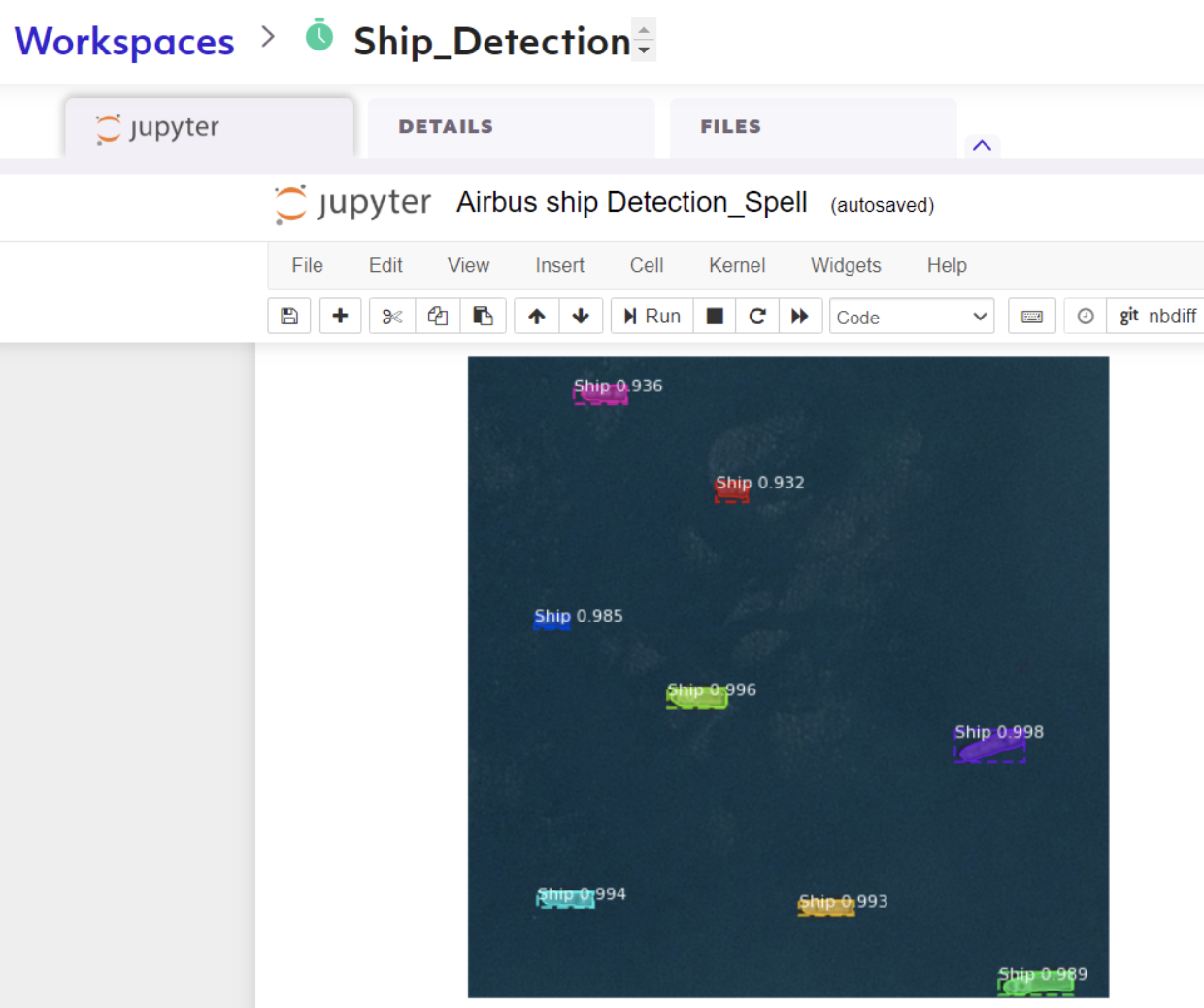On a successful run of code, results can be seen in notebook as shown above.

### Understanding the code

This is the case of the Instance Segmentation Computer Vision technique. The algorithm used for Instance segmentation is Mask R-CNN.

We have created a sample dataset with 55 train images and 19 test images. The output will be object detection in images with the label ship with their bounding boxes. Clone the Mask R-CNN repository like so:

``!git clone https://www.github.com/matterport/Mask_RCNN.git``

Change directory to Mask_RCNN and run the install script.

``````os.chdir('Mask_RCNN')
#!python setup.py -q install``````

Next we will prepare the dataset. We have 55 images for train data and 19 images for test data. We have all ship locations in images in the form of encoded pixels in ship_segmentations.csv file. Please find this sample dataset and CSV file and Jupyter notebook on GitHub.

``````marks1 = pd.read_csv('ship_segmentations.csv') # Markers for ships
marks1.reset_index(drop=True,inplace=True)
marks_unique = marks1[marks1.EncodedPixels.notnull()]

#Train data
marks=marks_unique[1:100]
images =list(marks.ImageId)

#Test data
test_marks=marks_unique[100:130]
test_images =list(test_marks.ImageId)

The data is in the following format: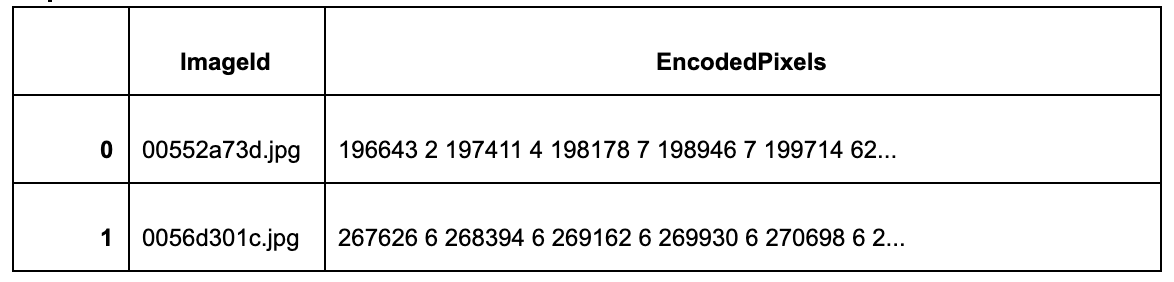Next, the code we will use to decode pixels:

``````def rle_decode(mask_rle, shape):
'''
mask_rle: run-length as string formated (start length)
shape: (height,width) of array to return
Returns numpy array, 1 - mask, 0 - background

'''
starts, lengths = [np.asarray(x, dtype=int) for x in (s[0:][::2], s[1:][::2])]
starts -= 1
ends = starts + lengths
img = np.zeros(shape*shape, dtype=np.uint8)
for lo, hi in zip(starts, ends):
img[lo:hi] = 1
return img.reshape(shape).T``````

``````class ShipDetection(Dataset):
"""Dataset class for training our dataset.
"""
# load the dataset definitions
def load_dataset(self, images_list, marks_ann, dataset_dir,orig_height, orig_width):
for i, fp in enumerate(images_list):
annotations=marks_ann.loc[marks_ann['ImageId'] == fp].EncodedPixels
self.add_image('ship', image_id=i, path=os.path.join(dataset_dir,  fp), anotations=annotations, orig_height=orig_height, orig_width=orig_width)

#Gives image info
def image_reference(self, image_id):
info = self.image_info[image_id]
print(info)
return info['path']

info=self.image_info[image_id]
#print(info)
# If grayscale. Convert to RGB for consistency.
if len(image.shape) != 3 or image.shape != 3:
image = np.stack((image,) * 3, -1)
return image

#print(self.image_info)
info=self.image_info[image_id]
annotations = info['annotations']
# print(image_id, annotations)
count = len(annotations)
if count == 0:
mask = np.zeros((info['orig_height'], info['orig_width'], 1), dtype=np.uint8)
class_ids = np.zeros((1,), dtype=np.int32)
else:
mask = np.zeros((info['orig_height'], info['orig_width'], count), dtype=np.uint8)
class_ids = np.zeros((count,), dtype=np.int32)
for i, a in enumerate(annotations):
mask[:, :, i] = rle_decode(a,SHAPE)
class_ids[i] = 1

Now we prepare our train and test datasets:

``````# prepare train set
train_set = ShipDetection()
train_set.prepare()

#Prepare test set
test_set = ShipDetection()
test_set.prepare()``````

Verify created datasets — let’s verify if datasets are created correctly or not.

``````# Test code to check if image loading, maskingworks properly on not
# load an image
image_id = 40

#display actual image and image with mask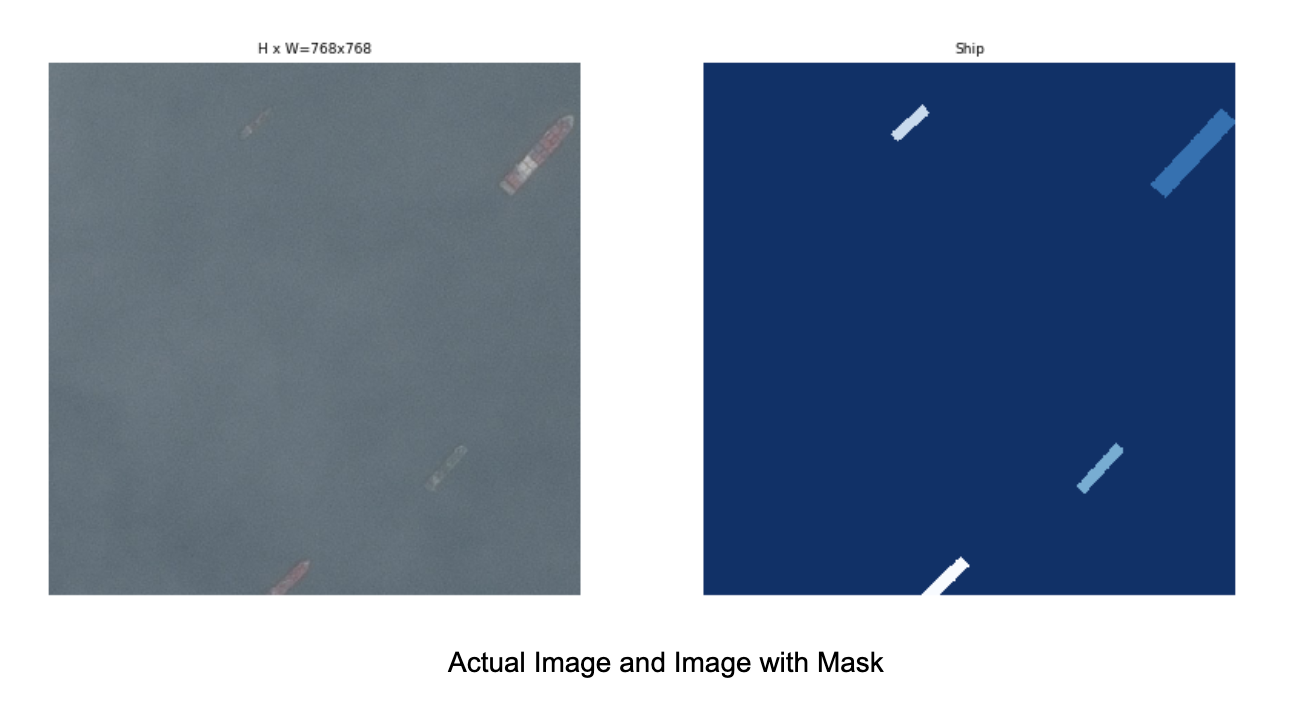It's finally time to train the model! We begin by defining an AirbusConfig class which extends the mrcnn.config.Config class.

``````class AirbusConfig(Config):
# Setting other parameters...

def __init__(self, num_classes):
self.NAME = "airbus_cfg"
self.NUM_CLASSES = num_classes
self.STEPS_PER_EPOCH =200
self.IMAGE_MAX_DIM=IMAGE_WIDTH
self.IMAGE_MIN_DIM=IMAGE_HEIGHT
super().__init__()

config = AirbusConfig(num_classes=2)
config.NUM_CLASSES
config.display()
``````

We define the model by creating an instance of the mrcnn.model.MaskRCNN class and set the mode argument to training using the configuration we defined above.

Finally, we load the pre-trained weights and train on them:

``````# define the model
model = MaskRCNN(mode='training', model_dir='./', config=config)
# load weights (mask_rcnn_coco) and exclude the output layers
# train weights (output layers or 'heads')
model.train(train_set, test_set, learning_rate=config.LEARNING_RATE, epochs=2, layers='heads')``````

A model is created by the end of every epoch and loss decreases with every epoch, so we will use the checkpoint from the second epoch, mask_rcnn_airbus_cfg_0002.h5, to evaluate model performance.

## Evaluating model performance

Now, it’s time to evaluate our model performance.

First, define the prediction configuration for evaluating the model

``````# Define the prediction configuration
class PredictionConfig(Config):
# Setting other parameters...
def __init__(self, num_classes):
self.NAME = "airbus_cfg"
self.NUM_CLASSES = 2
self.GPU_COUNT = 1
self.IMAGES_PER_GPU = 1
self.STEPS_PER_EPOCH=100
self.IMAGE_MAX_DIM=IMAGE_WIDTH
self.IMAGE_MIN_DIM=IMAGE_HEIGHT

super().__init__()

config_pred = PredictionConfig(2)
config_pred.display()``````

For prediction, set mode to inference and use the weights we got by training the model.

``````# define the model
model_eval = MaskRCNN(mode='inference', model_dir='./', config=config_pred)
# load model weights

Let’s evaluate the image and compare the actual image and predicted image.

``````def plot_actual_vs_predicted(dataset, model, cfg, image_id):

#Predicted image
scaled_image = mold_image(image, cfg)
# convert image into one sample
sample = expand_dims(scaled_image, 0)
# make prediction
yhat = model.detect(sample, verbose=0)

# Visualize results
r = yhat
display_instances(image, r['rois'], r['masks'], r['class_ids'],
dataset.class_names, r['scores'],title="Predicted",figsize=(8, 8))

# plot predictions for test dataset
plot_actual_vs_predicted(test_set, model_eval, config_pred,  image_id=1)

``````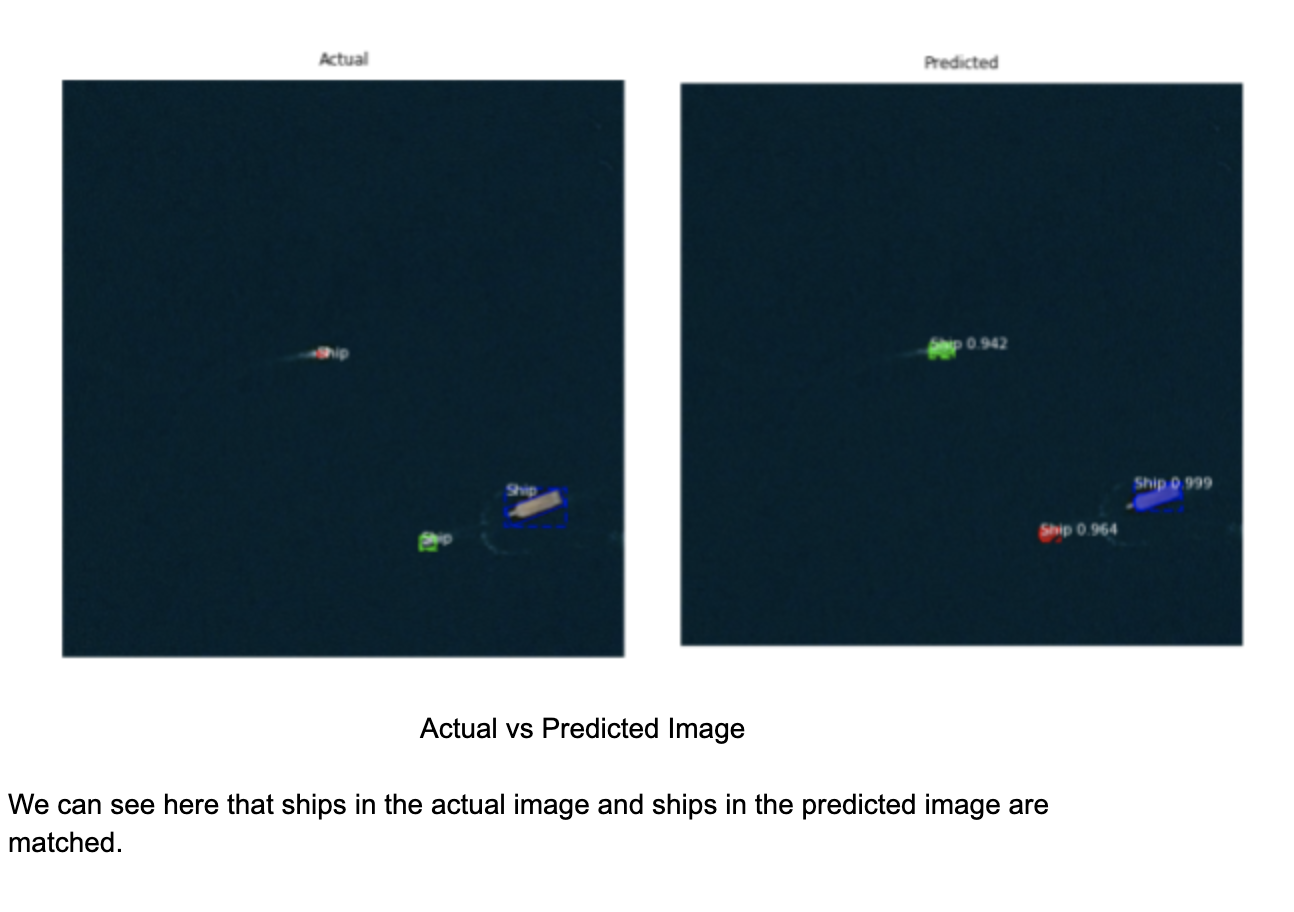Finally, let’s try to identify ships in new images (which are not used in train and test data).

``````def detect_cells(path):
plt.imshow(image)
# convert pixel values (e.g. center)
scaled_image = mold_image(image, config_pred)
# convert image into one sample
sample = expand_dims(scaled_image, 0)
# make prediction
yhat = model_eval.detect(sample, verbose=0)
r = yhat
display_instances(image, r['rois'], r['masks'], r['class_ids'],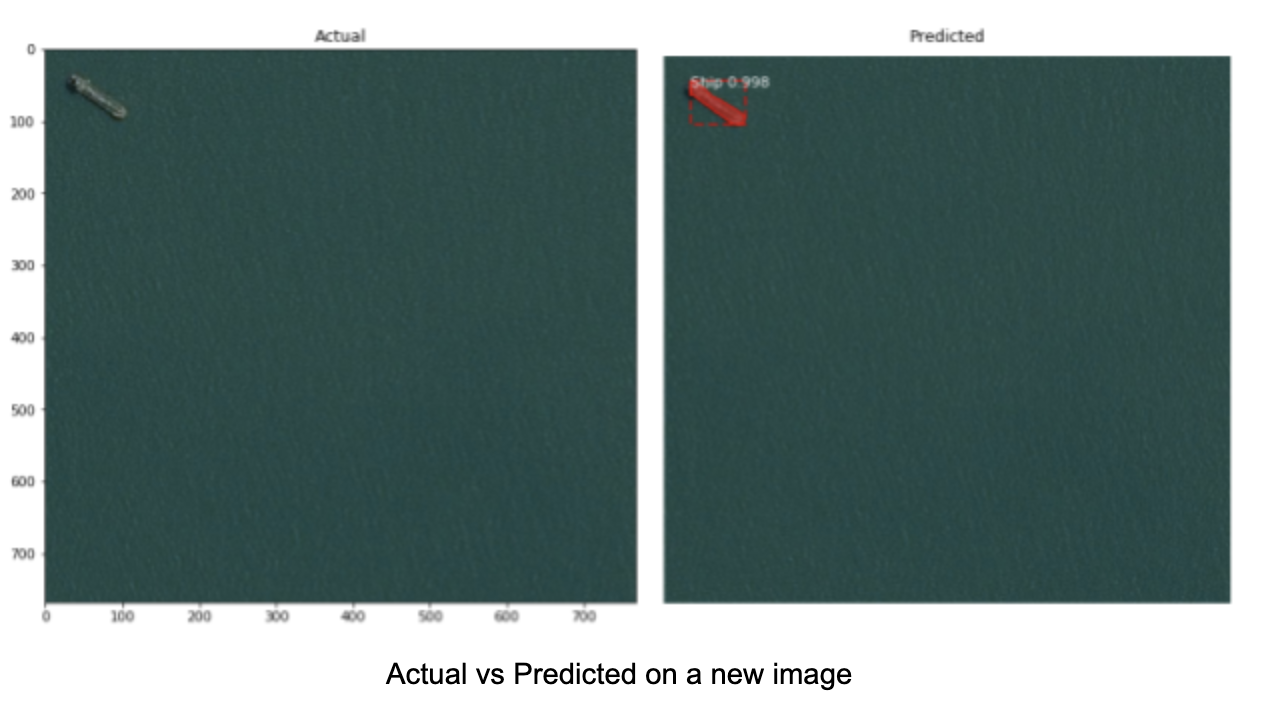Ships are detected in satellite images with good probability.

Let’s see a satellite image with multiple ships. This result predicted ships in a satellite image, and all eight ships were detected with greater than 0.9 probability.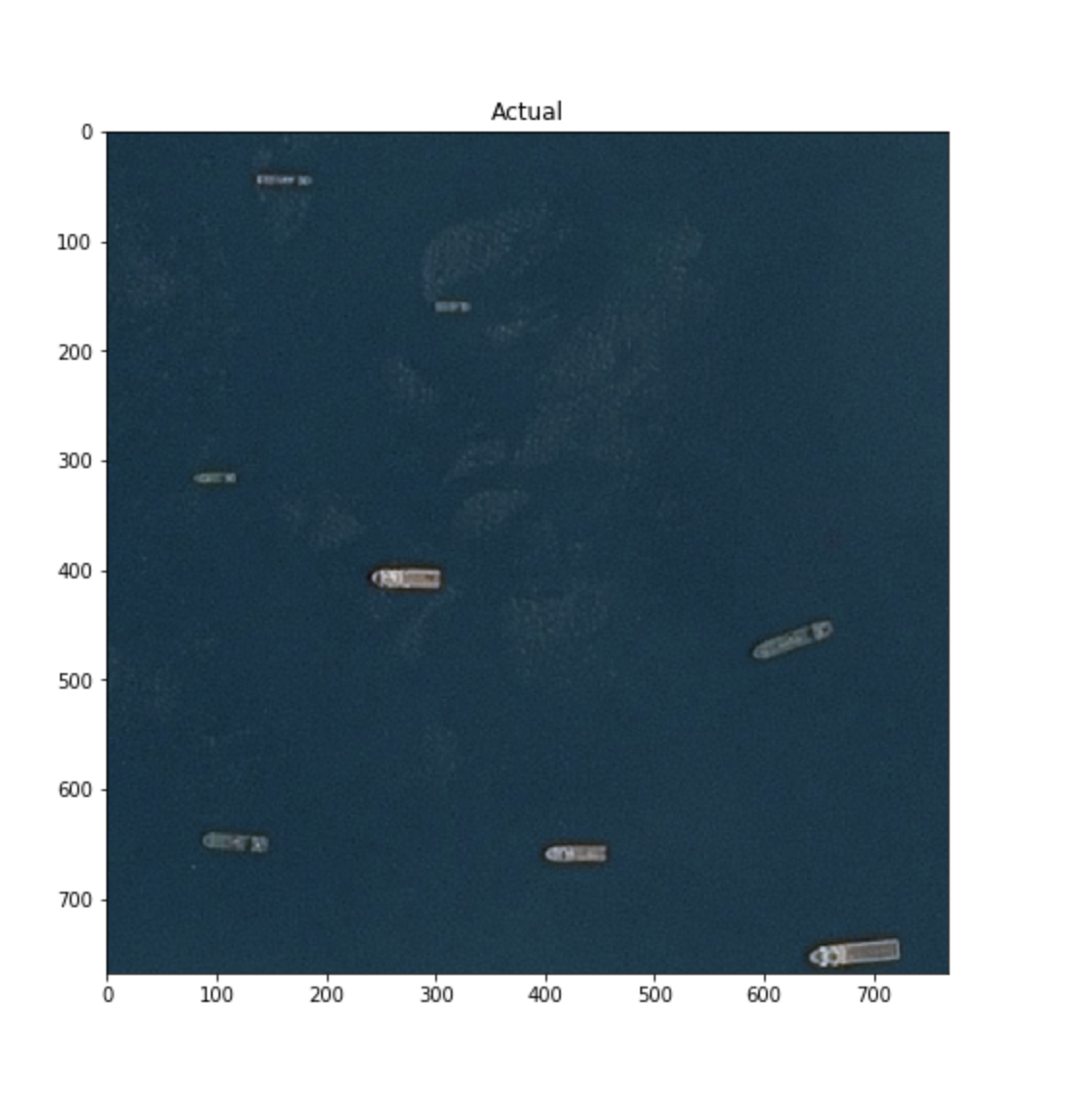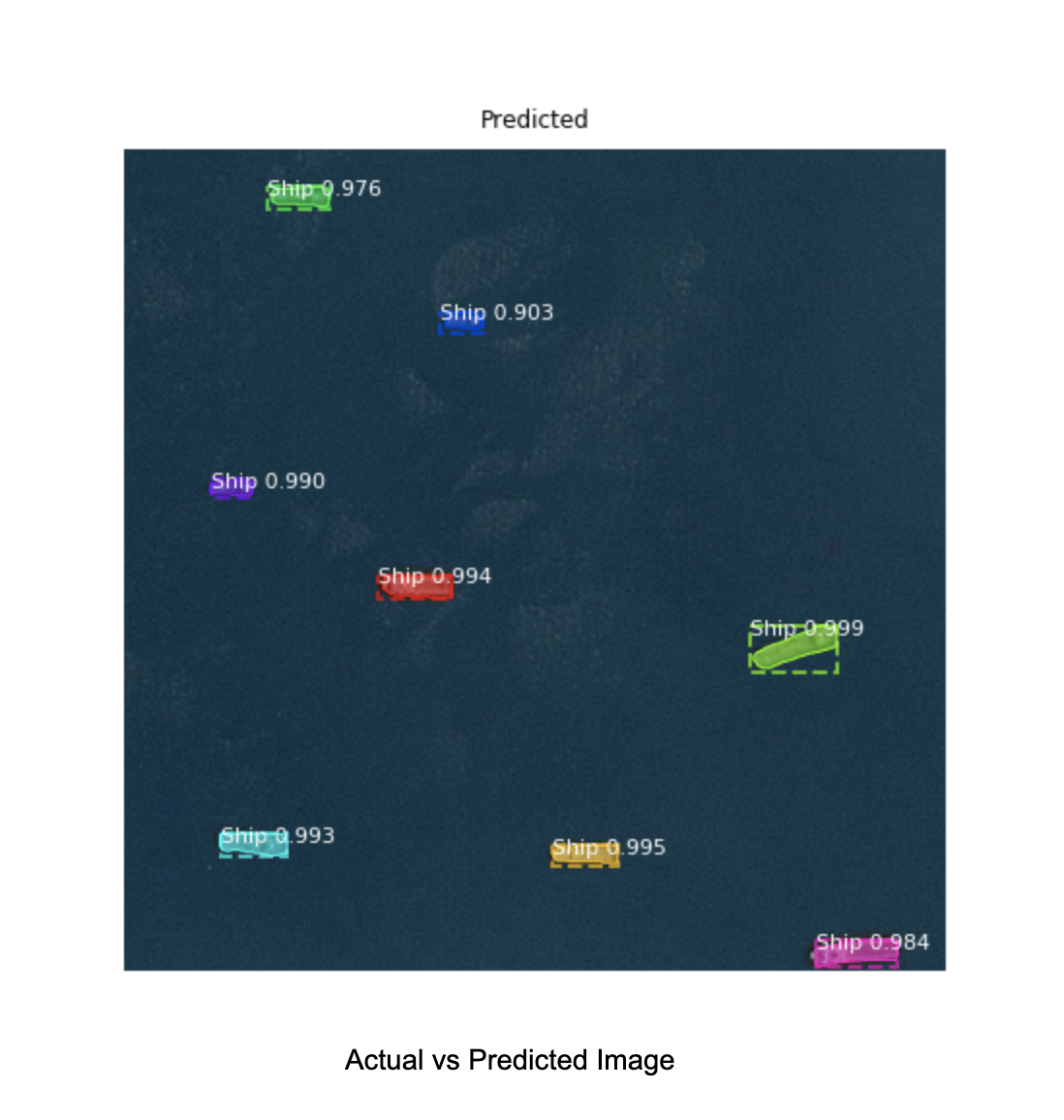## Conclusion

With Spell, the job is executed efficiently and the model works quite well, even with a smaller dataset You can explore this with larger datasets and accordingly increase the number of epochs and steps in epochs.

Spell’s ability to use GPUs allows us to easily dive into more deep learning problems not previously viable on regular machines with CPUs, making it a wonderful platform to explore and experiment with challenging machine learning problems.

Learn, implement, and enjoy machine learning with Spell.ml!

## Ready to Get Started?

Create an account in minutes or connect with our team to learn how Spell can accelerate your business.